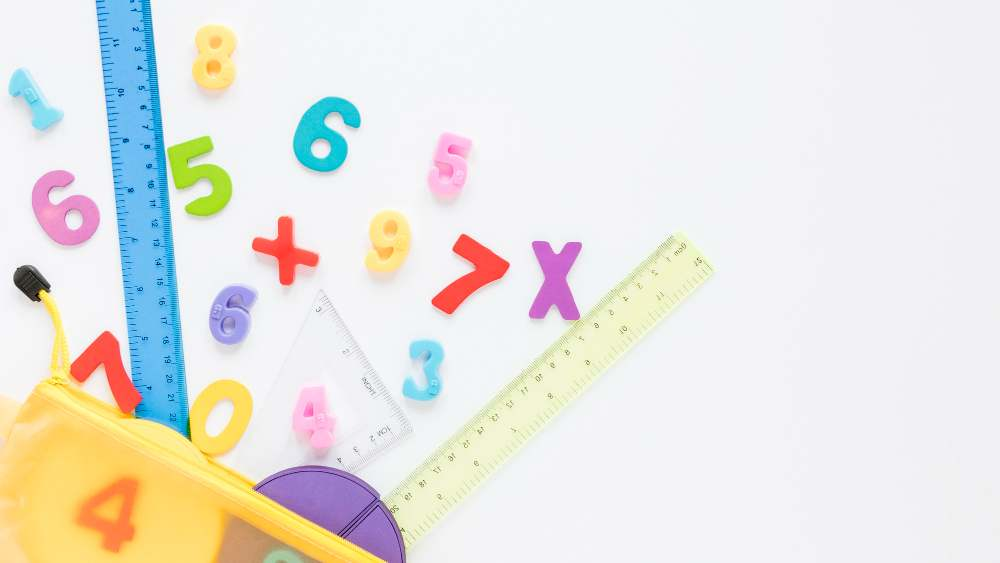Math concepts are the core of all mathematics. Every math problem, formula, equation that children encounter will have at least one math concept as its basis, especially in advanced mathematics.

Since children are bound to encounter math throughout their daily lives, understanding these basic math topics will give them a head start in their math learning journey.

In this blog, we’ll explore 7 mathematical concepts that children should be aware of in primary school. To give you an idea, these concepts include addition, subtraction, multiplication, division, fractions, and percentages!

Understanding these basic concepts early in their education will allow children to develop the confidence and expertise to tackle all kinds of math in the future, including maths concept exams!The first and most basic math concept that children should know is addition!

Addition is probably the easiest concept to understand. It simply involves combining the value of two or more numbers together, and you’re done!

When you encounter the plus symbol (+) in math equations, then you’ll know that you’re dealing with addition.

During the early years of primary school, children will tackle basic math addition questions, combining the total of numbers no higher than 100. Once children are accustomed to those numbers, only then will they add numbers in the hundreds and thousands.

Here are some common math questions using the addition concept:

• 7 + 19
• 38 + 12
• 40 + 20

If addition sounds intimidating to your child, don’t worry! You can ease them in by giving them simple and quick math quizzes to practice at home.

### 2) Subtraction

Meanwhile, the concept of subtraction is the complete opposite from addition.

Instead of combining two numbers together, subtraction involves taking away the value of a number. This means, subtraction is when you want to find the difference between two numbers.

You might recognize when you’re solving subtraction math questions if you see the “minus” symbol (-).

Here are some examples of math questions using subtraction:

• 10 – 8
• 39 – 21
• 93 – 12

### 3) Multiplication

Multiplication operates in a different field than addition and subtraction. But you might be surprised to know that it still involves the concept of addition.

Because multiplication involves repeatedly adding the same number several times.

But while you can manually add the numbers yourself, it’s more efficient to utilize the multiplication table for future math problems. The table includes the answers to every multiplication operation used from the numbers 1 to 10.

If your child has difficulties in memorizing the table, you can use some useful math tricks to make it easier for them!

The “times” symbol (x) in mathematics is used to represent multiplication. Note that the math symbol uses the lowercase of the letter “x”. You need to ensure that your child doesn’t mistake it with its capital counterpart!

Here are some multiplication math problems to give you an idea:

• 8 x 2
• 10 x 4
• 30 x 12

### 4) Division

If subtraction is the opposite of addition, then division is the opposite of multiplication.

Because while multiplication involves adding numbers repeatedly, division involves dividing a number into equal parts or groups.

Let’s imagine that you have several apples to sort into baskets. Multiplication is when you add the same number of apples repeatedly into a single basket. Meanwhile, division is when you spread out the apples into several baskets.

There are two symbols used in division, which are the “divide” symbol (÷) or the “slash” symbol (/). Each symbol has the same meaning, so it’s a matter of preference of when you use which symbol.

Here are some math questions using division:

• 42 / 7
• 25 / 5
• 12 / 4

### 5) Fractions

Now, here’s a math concept that’s completely different from the rest!

Fractions is a more difficult math concept to master. It involves dividing a whole number into several parts, but it also uses all of the math concepts we’ve discussed beforehand in its math equations.

You’ll recognize a fraction when you see two numbers divided by a single line, much like 12. The number above the line is called the “numerator” while the number below it is called the “denominator”.

You can also apply a math technique to understand fractions better!

Fractions essentially replace ordinary numbers in math problems. Here’s a few examples to illustrate it better:

• ½ + ¾
• ⅔ x ½
• 4 ÷ ½

You can also use video tutorials, including this video on comparing fractions, to learn more!

### 6) Percentages

The final math concept we’ll cover is the concept of percentages.

Much like multiplication involves addition, percentages also involve fractions, because a percentage is a fraction of 100 that represents a portion of a whole.

You’ll notice percentages because it has a distinct symbol, namely the “percentage” symbol (%). When you see a number using that symbol, it means that the number is divided by 100 – always.

Children will learn about this math concept in depth throughout primary school, often starting in Primary 2.

Here are some examples of percentages in math problems:

• 50% of 120
• 30% x 90
• 100 – 10%

### Learn math concepts with Practicle!

Learning these basic math concepts early will be a safety net for your children as they learn math at school.

You can also become more familiar with these concepts through constant revision, such as through Practicle’s math learning game!

Our gamified math app uses our own AI to personalise math questions based on each child’s learning level. They’re also delivered through fun math quests and challenges, making learning math a fun experience for children!

You can try the experience yourself using our 7-day free trial today!